## Example Questions

### Example Question #1 : Factoring

Two consecutive positive multiples of five have a product of 300. What is their sum?

35

25

45

20

15

35

Explanation:

Define the variables as x = 1st number and x + 5 = 2nd number, so the product is given as x(x + 5) = 300, which becomes x2 + 5x – 300 = 0.

Factoring results in (x + 20)(x – 15) = 0, so the positive answer is 15, making the second number 20.

The sum of the two numbers is 35.

### Example Question #2 : Factoring

Factor 12x3y+ 156x2y3

12x2y3(xy + 13)

x2y3(xy + 13)

12x2y3

12xy(xy + 13)

12x2y3(xy + 13)

Explanation:

The common factors are 12, x2, and y3.

So 12x2y3(xy + 13)

### Example Question #1 : How To Factor A Variable

Solve for all solutions of: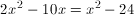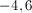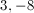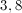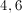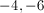Explanation:

First move all of the variables to the left side of the equation. Combine similar terms, and set the equation equal to zero. Then factor the equation to get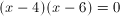Thus the solutions ofare 4 and 6.

### Example Question #4 : Factoring

Simplify: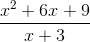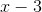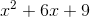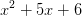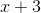Explanation:factors to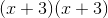One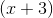cancels from the bottom, leaving### Example Question #5 : Factoring

Factor: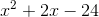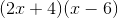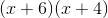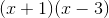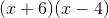Explanation:In the form of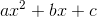you must find two numbers which add to give youand multiply to give youand then put them in the form of (+ number) (+ number)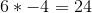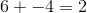Thereforeis the answer.

To check, multiply the two expressions out and it should equal### Example Question #6 : Factoring

Factor the following expression: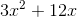The expression is already simplified as much as possible.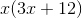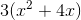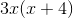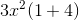Explanation:

To factor an expression we look for the greatest common factor.

Remember thatThus: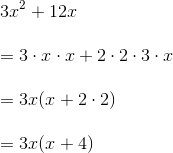### Example Question #7 : Factoring

Factor the following expression: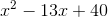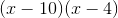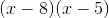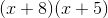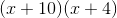Explanation:

To factor, you are looking for two factors of 40 that add to equal 13.

Factors of 40 include: (1, 40), (2, 20), (4, 10), (5, 8). Of these factors which two will add up to 13?

Also, since the first sign (-) and the second sign is (+) this tells us both binomials will be negative. This is because two negatives multiplied together will result in the positive third term, while two negatives added together will result in a larger negative number.

Thus,